# Hubert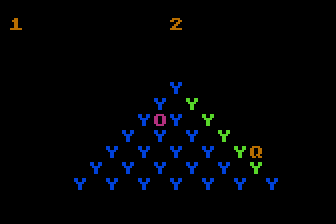This is a little game for the 2021 NOMAM's BASIC 10-liners Contest. This program fits in the "stock" PUR-80 category, and it was written in Atari BASIC for the 8-bits ATARI XL/XE. Development started on 2020-05-14, and it took 5 days. The final version's date is 2020-08-29.

UPDATE: It obtained the 8th place of 66 entries in the category.

## Description

Hubert has to change the color of the 5 pyramids to green, but Bally tries to make him fail the mission.

## InstructionsHubert starts in the top of the first pyramid. This is the only safe spot in it. Pyramid number appears in the upper-left corner, and at top appears the number of extra tries.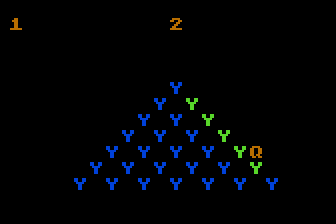Use your joystick to move Hubert though the pyramid. The joystick has to be rotated 45° to the right, i.e. you should use it with the red button centered on top.From time to time, an enemy called Bally will travel through the pyramid. Avoid him!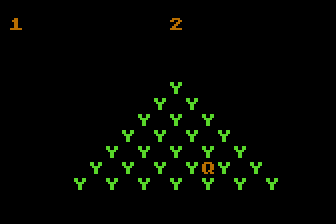Every time you reach a spot, its color might change. Try to change all the spots to green in order to complete the pyramid.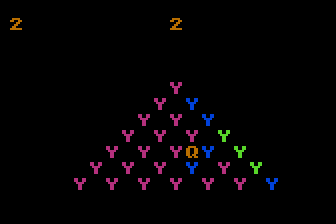While in the first pyramid you can get the green at the first jump in to it, in other pyramids you need to jump more times into every spot in order to meke them all green.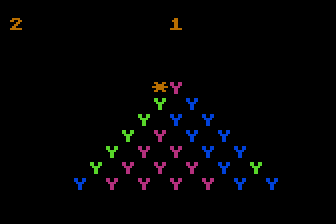Every time Bally gets Hubert, a try is lost. Bally will increase his speed and frecuency as time runs.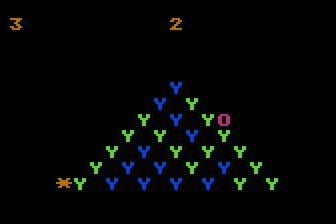If you make Hubert jump out of the pyramit, you will also loose a try, and all the work already done in that pyramid will be lost.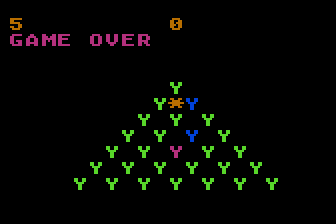When there are no more tries, the mission has failed and the game is over. Press the button to try again.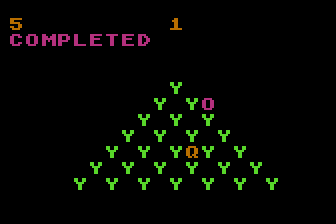You win only when all 5 pyramids were converted to green ones.

## Development of the game

I've always liked the Q*Bert character, and decided to program his game for the tenliners contest. I started with a simple pyramid design in graphics mode 12 (ANTIC mode 4) in order to test how it would look and if I could manage all of its states in TurboBASIC XL or Fastbasic. I started coding a prototype in Fastbasic, because I also designed the sprite for Q*Bert and I wanted to see him moving through the pyramid. I also found a formula for the color transitions of the cubes, 5 different sequences for that amount of levels, and it looked great.

I was surprised that the code to make the character move through the pyramid without smooth transitions between spots was very simple, so I rewote it in Atari BASIC to measure how much space it would require. Of course, I ignored the bitmaps and sprites, and selected the text mode 2 (ANTIC mode 7) to display the pyramid and the character.

It seemed to work very good, and I had to decide what to do with the few extra space I had. Options were two: (1) modify the charset and change the graphics into bitmaps, or (2), add an opponent and make the game challenging and enjoyable. I went for option (2).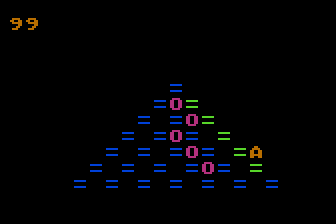Prototype with a moving opponent (with trail)

I managed to add sound effects and limited the game to just those 5 different levels, which became the target of this variation. This turned into a mission without score, just few lives, which are lost when the opponent catches Hubert or Hubert falls out of the pyramid, and uncompleated pyramids are reset to try again. Also, I tried different combination of letters and sysmbols to draw the playfield.

As this finished game it is a simplistic one, I just wanted to name it like the suggested name for the original game: "Hubert". By the time I'm writting this, I hope to resume the original Q*Bert project with P/M sprites. I took a break to build a device which would allow me to connect a real Atary CX40 joystick to my PC and test these Q*Bert series of games in the emulator using it, instead of emulating it with the keyboard that it cannot be rotated 45°.

Get the HUBERT.ATR file and set it as drive 1 in a real Atari (or emulator). Turn on the computer and the game should start after loading. A joystick in port 1 is required, and it has to be rotated 45° clockwise to play.

## The code

The abbreviated BASIC code is the following: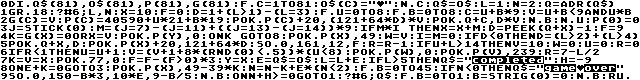The full and expanded BASIC listing is:

 Hubert (c) 2020 Víctor Parada 2020-08-29 GR.18 scr: 112,158 = 40560 Pyramid's top pointer (for player): 40590 (40560+20*2+10) ```0 ``` LINE 0: Initialization ```dim q\$(81),o\$(81),p(81),g(81) ``` Q\$: Current status of each cube O\$: Required status of each cube P(): Pointer to a screen position of each cube G(): Valid cubes ```for c=1 to 81 o\$(c)="{zero}" next c q\$=o\$ ``` Initialize strings ```l=1 ``` Level ```n=2 ``` Remaining (extra) Lives ```q=adr(q\$) ``` Status of cubes Other variables auto-initilized at run Y=0: last position of Hubert I=0: Joystick is in motion state R=0: Enemy's response timer ```1 ``` LINE 1: LEVEL LOOP Initialize level ```graphics 18 ``` Sets graphics mode 2+16 (20x12 text mode with 4 colors plus background) ```print #6;l,n ``` Prints current level (in the upper-left corner) and the number of remaining lives (at the center of the top) ```x=10 ``` Starting position of Hubert (top of the pyramid) ```f=0 ``` Reset FX ```d=1+(l>1)-(l=3) ``` Sets starting color for level ```for u=0 to 8 for b=0 to 8 ``` Iterates over the matrix ``` c=u+b*9 ``` Gets the sequential pointer ``` v=u+b<9 and u*b ``` Determines if the pointer is part of the pyramid ```2 ``` LINE 2: Level linitialization (cont) ``` g(c)=v ``` Saves the status of the pointer ``` p(c)=40590+u*21+b*19 ``` Maps the pointer into screen ``` poke p(c)+20,(121+64*d)*v ``` Puts a cube in the screen if it's part of the pyramid ``` poke q+c,d*v ``` Marks the initial status of a cube ``` next b next u ``` Continues loop ```p(0)=0 ``` Sets the default off-the-piramid pointer out of screen At the end of the initialization U=9: Enemy's counter V=0: Position of the Enemy ```3 ``` LINE 3: PLAYING LOOP ```j=stick(0) m=(j=7)-(j=11)+((j=13)-(j=14))*9 ``` Joystick was moved? ```if m*i then x=x+m ``` If joystick was just moved, find the new position ``` d=peek(q+x)-1 ``` Gets the next status of the new cube ``` f=9 ``` Enables jumping sound FX ```4 ``` LINE 4: Relocates Hubert ```k=g(x)=0 or x=v ``` Checks if Hubert fall out of the pyramid or if it crashed with the enemy ```poke p(y),0 ``` Removes Hubert from the screen ```on k goto 8 ``` Quit playing loop if killed ```poke p(x),49 ``` Puts Hubert in the new cube of the pyramid ```w=v ``` Saves enemy's old position ```i=m=0 ``` Verifies that joystick was previously released ```if d<0 then d=(l>2)+(l>4) ``` Fixes next cube's color in level's cycle ```5 ``` LINE 5: Updates current cube ```poke q+x,d ``` Updates cube in status ```poke p(x)+20,121+64*d ``` Updates cube ```sound 0,161,12,f ``` Jumping FX ```r=r-1 ``` Decreases enemy response timer ```if u+l>14 then v=10 w=0 u=0 r=0 ``` Triggers the enemy ```6 ``` LINE 6: Moving the enemy ```if r<1 then u=u+1 ``` Move enemy? ``` v=(v+1+8*(rnd(0)<.5))*(u<8) ``` Calculates next position ``` poke p(w),0 ``` Remove from latest position ``` poke p(v),239 ``` Puts enemy in new position ``` r=7-l/2 ``` Resets the enemy response timer ```7 ``` LINE 7: Completes the playing loop ```k=v=x ``` Checks if enemy hit Hubert ```poke 77,0 ``` Disables attract mode ```f=f-(f>0)*3 ``` Next step for the jump FX ```y=x ``` Save Hubert's old position ```e=q\$=o\$ ``` Completed? ```l=l+e ``` Increases level if completed ```if l>5 then q\$="COMPLETED" ``` No more levels? ``` h=-9 ``` Forces end of game ```8 ``` LINE 8: Checks for the end of the level ```on e+k=0 goto 3 ``` Repeat playing loop until killed or completed level ```poke p(x),49-39*k ``` Update Hubert ```n=n-k+e*(n<2) ``` Decrease lifes if killed or increase up to the maximum if survived ```for b=0 to 45 ``` Performs kill or end of level tune ``` if n<0 then q\$="GAME OVER" ``` No more lives? Code placed inside the loop just to fit the line length restriction ```9 ``` LINE 9: End of level/game ``` sound 0,150-b*3,10*e,9-b/5 next b ``` ```on n+h>=0 goto 1 ``` Repeat or start new level ```print #6;q\$ ``` ```for b=0 to 1 b=strig(0)=0 next b ``` Waits for the trigger ```run ``` Restarts